Related Articles

# Class 8 RD Sharma Solutions – Chapter 19 Visualising Shapes – Exercise 19.2

• Last Updated : 12 Mar, 2021

### Question 1. Which of the following are nets for a cube?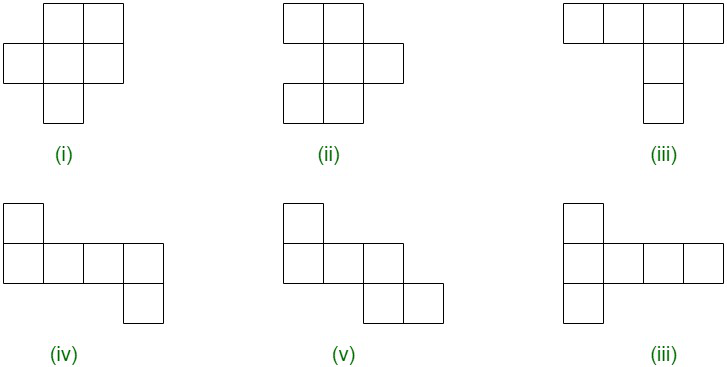Solution:

Diagram (iv), (v), (vi) are the nets for a cube.

### Question 2. Name the polyhedron that can be made by folding each net: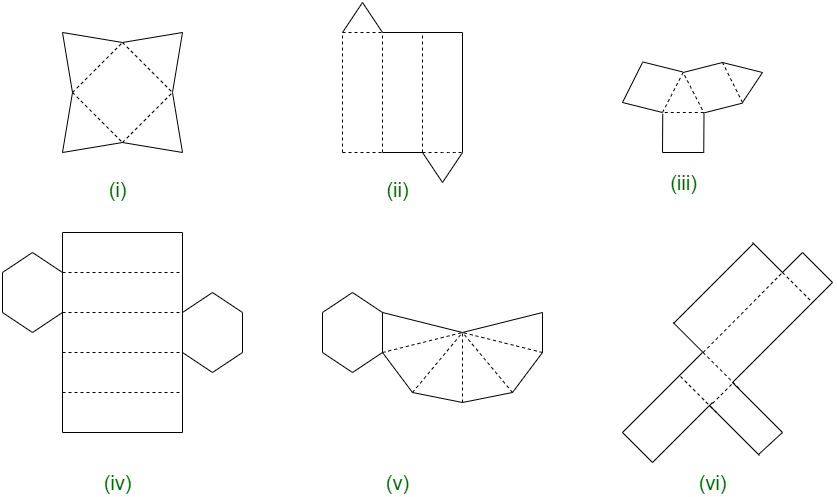Solution:

(i) By folding each net a square pyramid can be formed.

(ii) By folding each net a triangular prism can be formed.

(iii) By folding each net a triangular prism can be formed.

(iv) By folding each net a hexagonal prism can be formed.

(v) By folding each net a hexagonal prism can be formed.

(vi) By folding each net a Cuboid can be formed.

### Question 3. Dice are cubes where the numbers on the opposite faces must total 7. Which of the following are dice?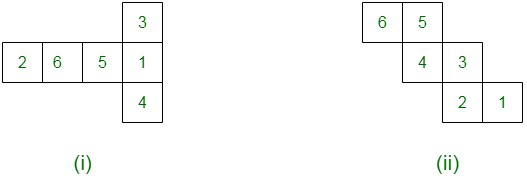Solution:

The Figure (i) form a dice and the total sum of numbers on opposite faces is 7.

### Question 4. Draw nets for each of the following polyhedrons: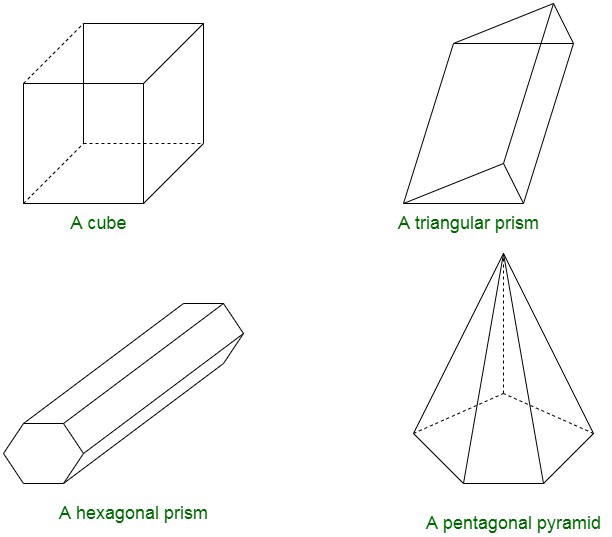Solution:

(i) Below image shows the net pattern for cube.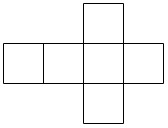(ii) Below image shows the pattern for triangular prism.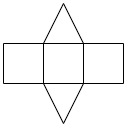(iii) Below image shows the net pattern for hexagonal prism.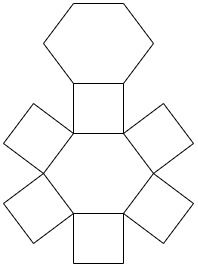(iv) Below image shows the net pattern for pentagonal pyramid.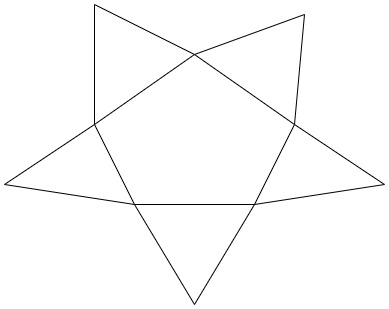### Question 5. Match the following figures: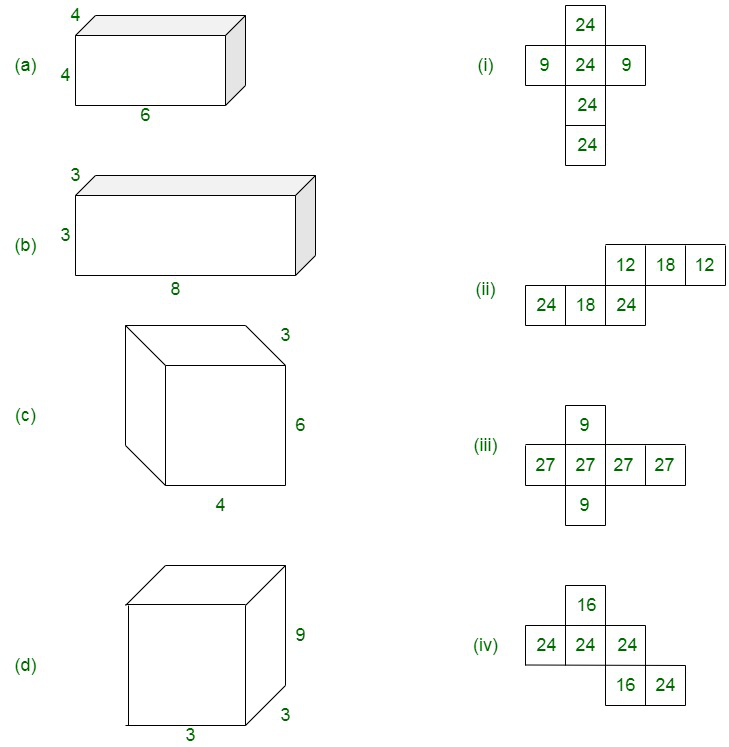Solution:

(a) ⇒ (iv)

Here, the multiplication of numbers on adjacent faces are equal, i.e., 6×4 = 24 and 4×4 = 16

(b) ⇒ (i)

Here, the multiplication of numbers on adjacent faces are equal, i.e., 3×3 = 9 and 8×3 = 24

(c) ⇒ (ii)

Here, the multiplication of numbers on adjacent faces are equal, i.e., 6×4 = 24 and 6×3 = 18

(d) ⇒ (iii)

Here, the multiplication of numbers on adjacent faces are equal, i.e., 3×3 = 9 and 3×9 = 7

Attention reader! Don’t stop learning now. Participate in the Scholorship Test for First-Step-to-DSA Course for Class 9 to 12 students.

My Personal Notes arrow_drop_up